#### Chapter 3 Rationalization R.D. Sharma Solutions for Class 9th Math Exercise 3.1

Exercise 3.1

1. Simplify each of the following
(i)(ii)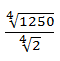Solution

(i)(ii)2. Simplify the following expressions.
(i)(ii)(iii)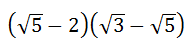Solution

(i)(ii)(iii)3. Simplify the following expressions :
(i)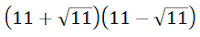(ii)(iii)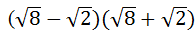(iv)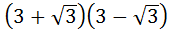(v)Solution

(i)(ii)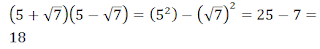(iii)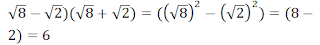(iv)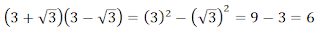(v)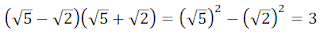4. Simplify the following expressions :
(i)(ii)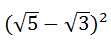(iii)Solution

(i)(ii)(iii)